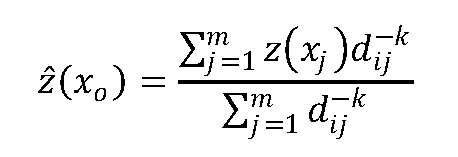## Name

ST_InvDistWeight4ma — Raster processing function that interpolates a pixel's value from the pixel's neighborhood.

## Synopsis

`double precision ST_InvDistWeight4ma(`double precision[][][] value, integer[][] pos, text[] VARIADIC userargs`)`;

## Description

Calculate an interpolated value for a pixel using the Inverse Distance Weighted method.

There are two optional parameters that can be passed through `userargs`. The first parameter is the power factor (variable k in the equation below) between 0 and 1 used in the Inverse Distance Weighted equation. If not specified, default value is 1. The second parameter is the weight percentage applied only when the value of the pixel of interest is included with the interpolated value from the neighborhood. If not specified and the pixel of interest has a value, that value is returned.

The basic inverse distance weight equation is:k = power factor, a real number between 0 and 1This function is a specialized callback function for use as a callback parameter to ST_MapAlgebra (callback function version).

Availability: 2.1.0

## Examples

```-- NEEDS EXAMPLE
```